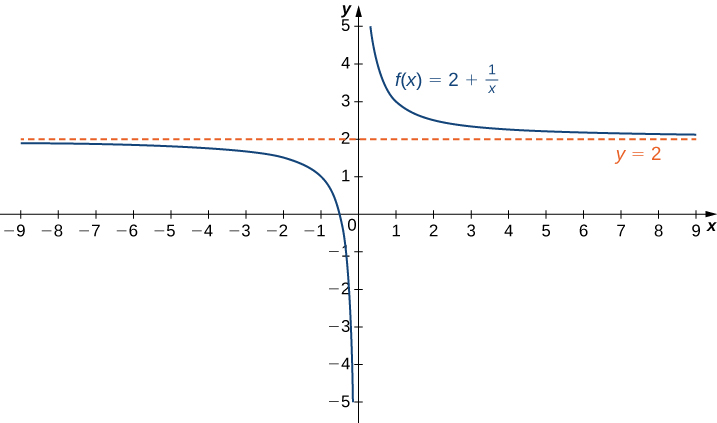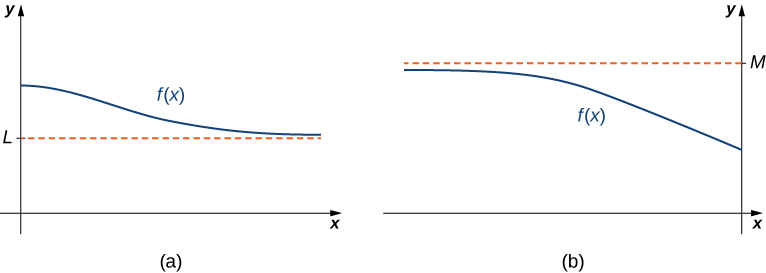# 4.6 Limits at infinity and asymptotes

 Page 1 / 14
• Calculate the limit of a function as $x$ increases or decreases without bound.
• Recognize a horizontal asymptote on the graph of a function.
• Estimate the end behavior of a function as $x$ increases or decreases without bound.
• Recognize an oblique asymptote on the graph of a function.
• Analyze a function and its derivatives to draw its graph.

We have shown how to use the first and second derivatives of a function to describe the shape of a graph. To graph a function $f$ defined on an unbounded domain, we also need to know the behavior of $f$ as $x\to \text{±}\infty .$ In this section, we define limits at infinity and show how these limits affect the graph of a function. At the end of this section, we outline a strategy for graphing an arbitrary function $f.$

## Limits at infinity

We begin by examining what it means for a function to have a finite limit at infinity. Then we study the idea of a function with an infinite limit at infinity. Back in Introduction to Functions and Graphs , we looked at vertical asymptotes; in this section we deal with horizontal and oblique asymptotes.

## Limits at infinity and horizontal asymptotes

Recall that $\underset{x\to a}{\text{lim}}f\left(x\right)=L$ means $f\left(x\right)$ becomes arbitrarily close to $L$ as long as $x$ is sufficiently close to $a.$ We can extend this idea to limits at infinity. For example, consider the function $f\left(x\right)=2+\frac{1}{x}.$ As can be seen graphically in [link] and numerically in [link] , as the values of $x$ get larger, the values of $f\left(x\right)$ approach $2.$ We say the limit as $x$ approaches $\infty$ of $f\left(x\right)$ is $2$ and write $\underset{x\to \infty }{\text{lim}}f\left(x\right)=2.$ Similarly, for $x<0,$ as the values $|x|$ get larger, the values of $f\left(x\right)$ approaches $2.$ We say the limit as $x$ approaches $\text{−}\infty$ of $f\left(x\right)$ is $2$ and write $\underset{x\to a}{\text{lim}}f\left(x\right)=2.$The function approaches the asymptote y = 2 as x approaches ± ∞ .
 $x$ $10$ $100$ $1,000$ $10,000$ $2+\frac{1}{x}$ $2.1$ $2.01$ $2.001$ $2.0001$ $x$ $-10$ $-100$ $-1000$ $-10,000$ $2+\frac{1}{x}$ $1.9$ $1.99$ $1.999$ $1.9999$

More generally, for any function $f,$ we say the limit as $x\to \infty$ of $f\left(x\right)$ is $L$ if $f\left(x\right)$ becomes arbitrarily close to $L$ as long as $x$ is sufficiently large. In that case, we write $\underset{x\to a}{\text{lim}}f\left(x\right)=L.$ Similarly, we say the limit as $x\to \text{−}\infty$ of $f\left(x\right)$ is $L$ if $f\left(x\right)$ becomes arbitrarily close to $L$ as long as $x<0$ and $|x|$ is sufficiently large. In that case, we write $\underset{x\to \text{−}\infty }{\text{lim}}f\left(x\right)=L.$ We now look at the definition of a function having a limit at infinity.

## Definition

(Informal) If the values of $f\left(x\right)$ become arbitrarily close to $L$ as $x$ becomes sufficiently large, we say the function $f$ has a limit at infinity    and write

$\underset{x\to \infty }{\text{lim}}f\left(x\right)=L.$

If the values of $f\left(x\right)$ becomes arbitrarily close to $L$ for $x<0$ as $|x|$ becomes sufficiently large, we say that the function $f$ has a limit at negative infinity and write

$\underset{x\to \infty }{\text{lim}}f\left(x\right)=L.$

If the values $f\left(x\right)$ are getting arbitrarily close to some finite value $L$ as $x\to \infty$ or $x\to \text{−}\infty ,$ the graph of $f$ approaches the line $y=L.$ In that case, the line $y=L$ is a horizontal asymptote of $f$ ( [link] ). For example, for the function $f\left(x\right)=\frac{1}{x},$ since $\underset{x\to \infty }{\text{lim}}f\left(x\right)=0,$ the line $y=0$ is a horizontal asymptote of $f\left(x\right)=\frac{1}{x}.$

## Definition

If $\underset{x\to \infty }{\text{lim}}f\left(x\right)=L$ or $\underset{x\to \text{−}\infty }{\text{lim}}f\left(x\right)=L,$ we say the line $y=L$ is a horizontal asymptote    of $f.$(a) As x → ∞ , the values of f are getting arbitrarily close to L . The line y = L is a horizontal asymptote of f . (b) As x → − ∞ , the values of f are getting arbitrarily close to M . The line y = M is a horizontal asymptote of f .

how does this work
Can calculus give the answers as same as other methods give in basic classes while solving the numericals?
log tan (x/4+x/2)
Rohan
Rohan
y=(x^2 + 3x).(eipix)
Claudia
Ismael
A Function F(X)=Sinx+cosx is odd or even?
neither
David
Neither
Lovuyiso
f(x)=1/1+x^2 |=[-3,1]
apa itu?
fauzi
determine the area of the region enclosed by x²+y=1,2x-y+4=0
Hi
MP
Hi too
Vic
hello please anyone with calculus PDF should share
Which kind of pdf do you want bro?
Aftab
hi
Abdul
can I get calculus in pdf
Abdul
How to use it to slove fraction
Hello please can someone tell me the meaning of this group all about, yes I know is calculus group but yet nothing is showing up
Shodipo
You have downloaded the aplication Calculus Volume 1, tackling about lessons for (mostly) college freshmen, Calculus 1: Differential, and this group I think aims to let concerns and questions from students who want to clarify something about the subject. Well, this is what I guess so.
Jean
Im not in college but this will still help
nothing
how can we scatch a parabola graph
Ok
Endalkachew
how can I solve differentiation?
with the help of different formulas and Rules. we use formulas according to given condition or according to questions
CALCULUS
For example any questions...
CALCULUS
v=(x,y) وu=(x,y ) ∂u/∂x* ∂x/∂u +∂v/∂x*∂x/∂v=1
log tan (x/4+x/2)
Rohan
what is the procedures in solving number 1?
review of funtion role?
for the function f(x)={x^2-7x+104 x<=7 7x+55 x>7' does limx7 f(x) exist?
find dy÷dx (y^2+2 sec)^2=4(x+1)^2
Integral of e^x/(1+e^2x)tan^-1 (e^x)
why might we use the shell method instead of slicing
fg[[(45)]]²+45⅓x²=100By Brooke DelaneyBy Steve GibbsBy OpenStaxBy Madison ChristianBy Michael SagBy OpenStaxBy Yacoub JayoghliBy OpenStaxBy Rohini AjayBy Yasser Ibrahim